# NCERT Solutions for Class 9 Science Chapter 8: Motion

## NCERT Solutions Class 9 Science Chapter 8 – CBSE Term I Free PDF Download

NCERT Solutions for Class 9 Science Chapter 8 Motion is designed with the intention of clarifying doubts and concepts easily. Class 9 solutions in science is a beneficial reference and guiding solution that helps students clear doubts instantly, in an effective way.

NCERT Solutions for Class 9 Science approaches students in a student-friendly way and is loaded with questions, activities, and exercises that are CBSE Term I exam and competitive exam-oriented. NCERT Solutions for Class 9 Science is the contribution by the faculty having vast teaching experience. It is developed keeping in mind the concept-based approach along with the precise answering method for CBSE Term I examinations. Refer NCERT Solutions for Class 9 for best scores in CBSE Term I and competitive exams. It is a detailed and well-structured solution for a solid grip of the concept-based learning experience. NCERT for Class 9 Science Solutions are made available in both web and PDF format for ease of access.

## Download PDF of NCERT Solutions for Class 9 Science Chapter 8: Motion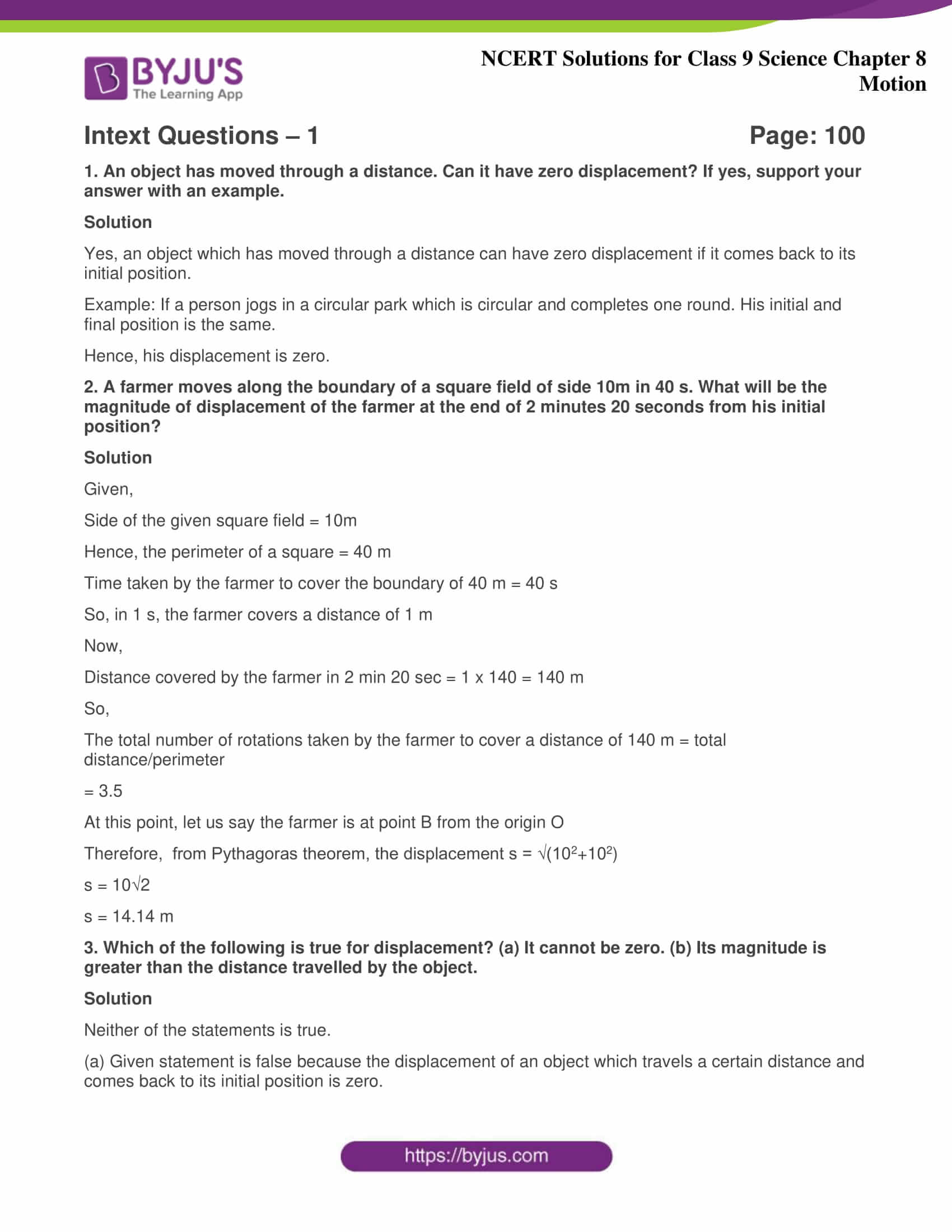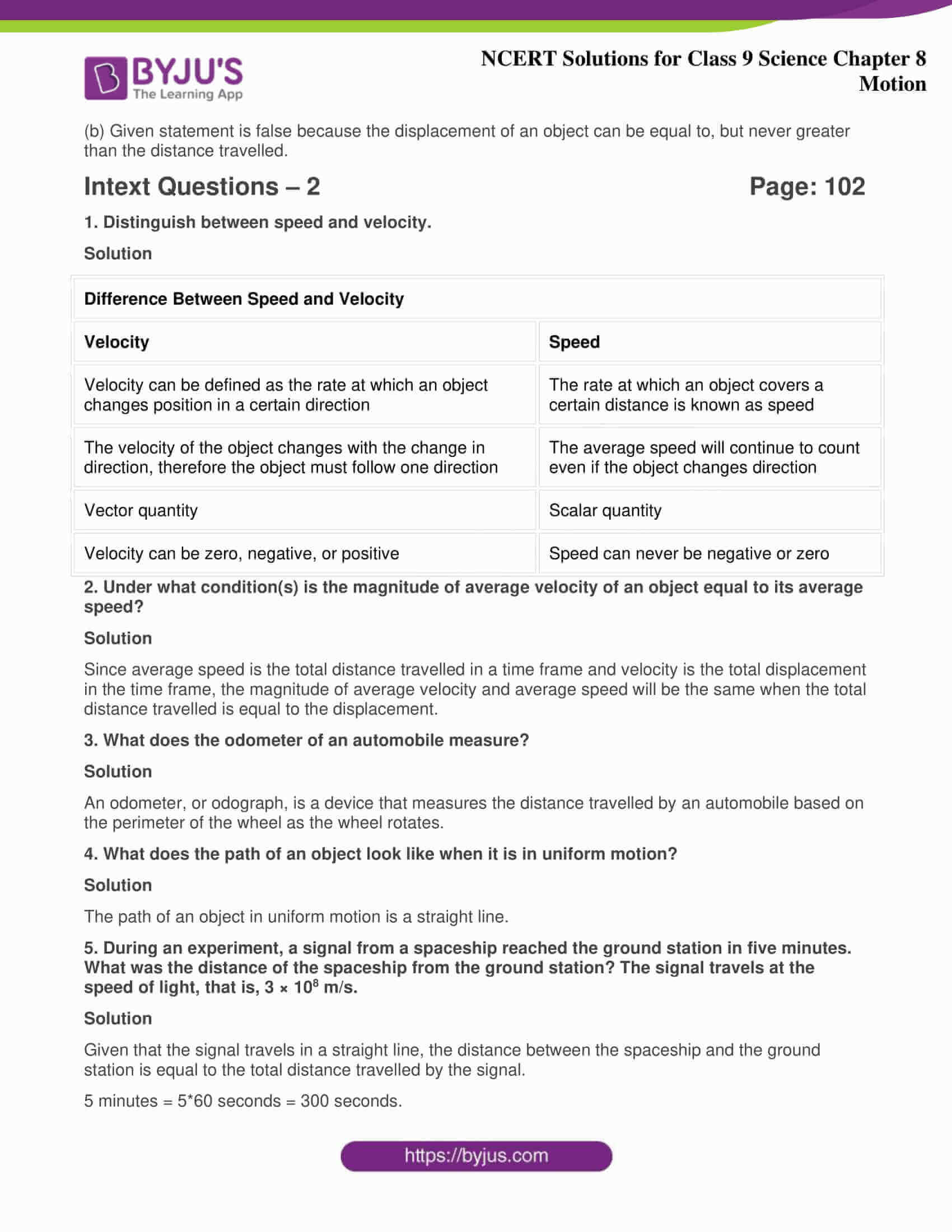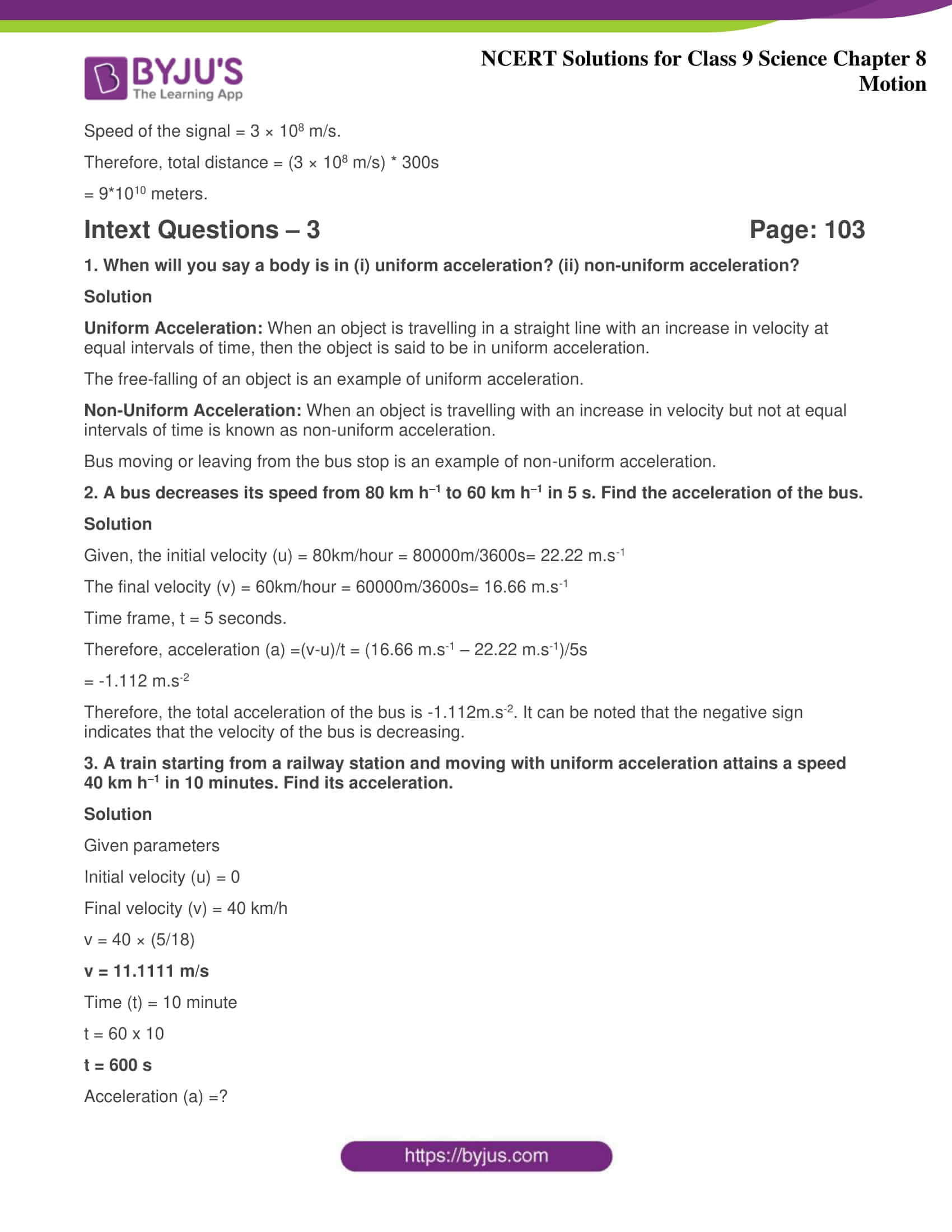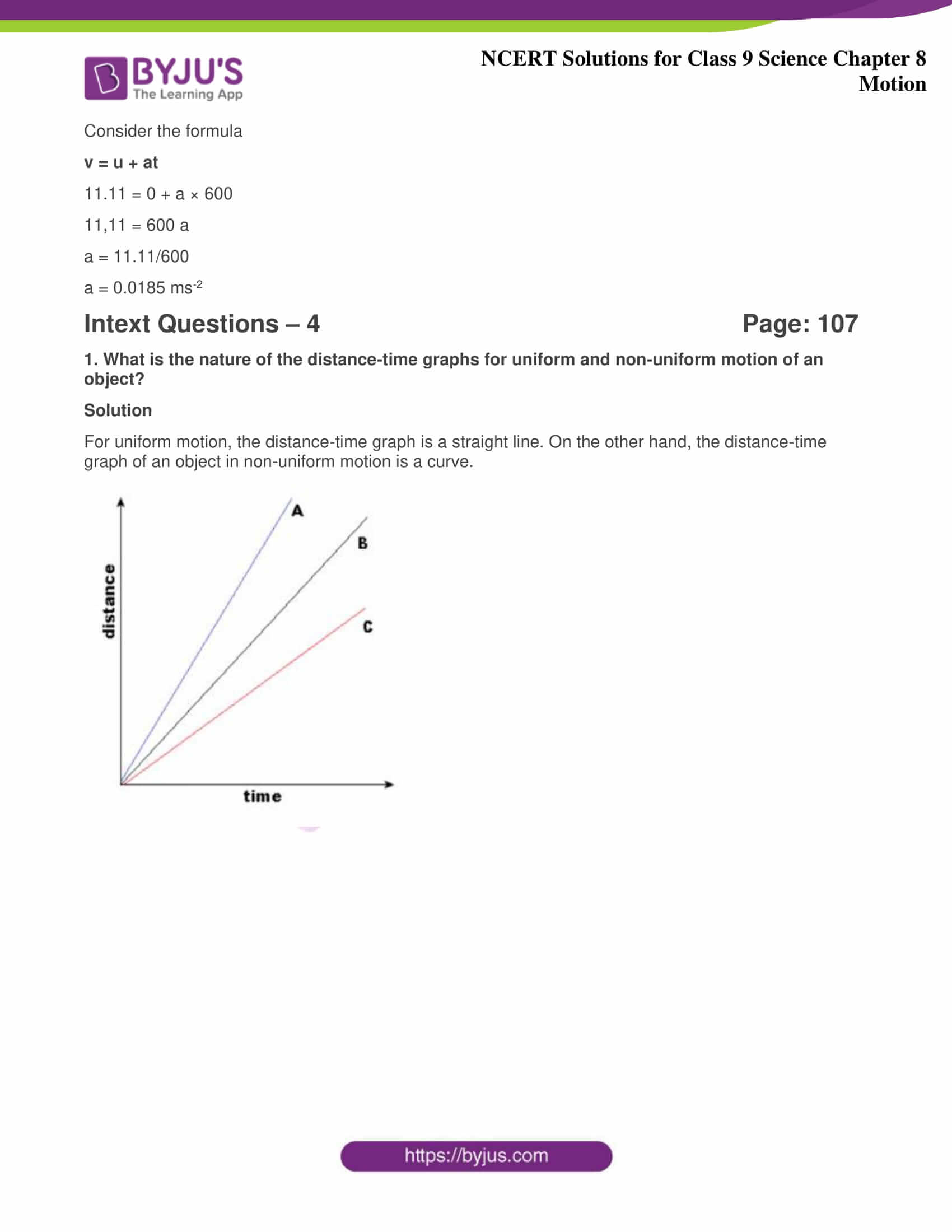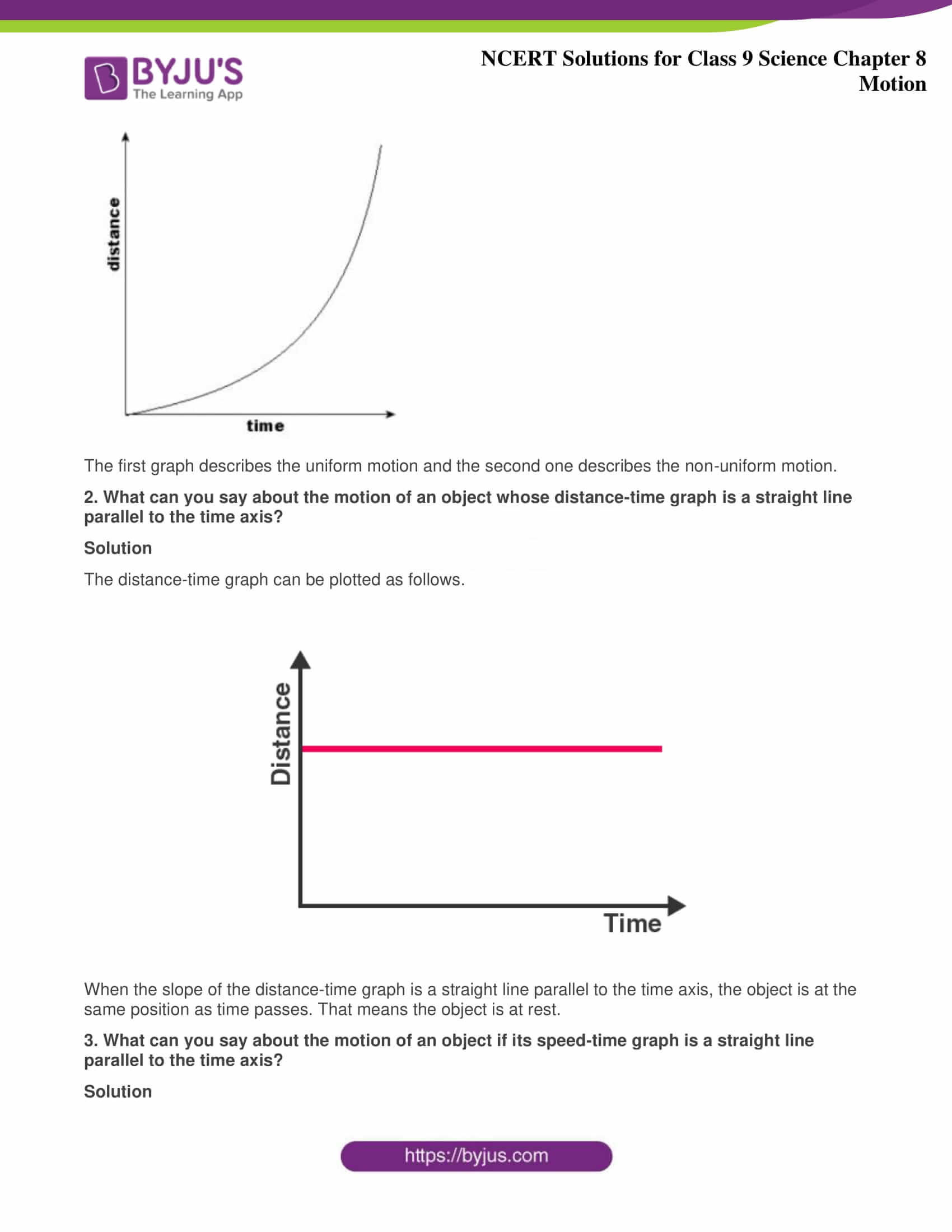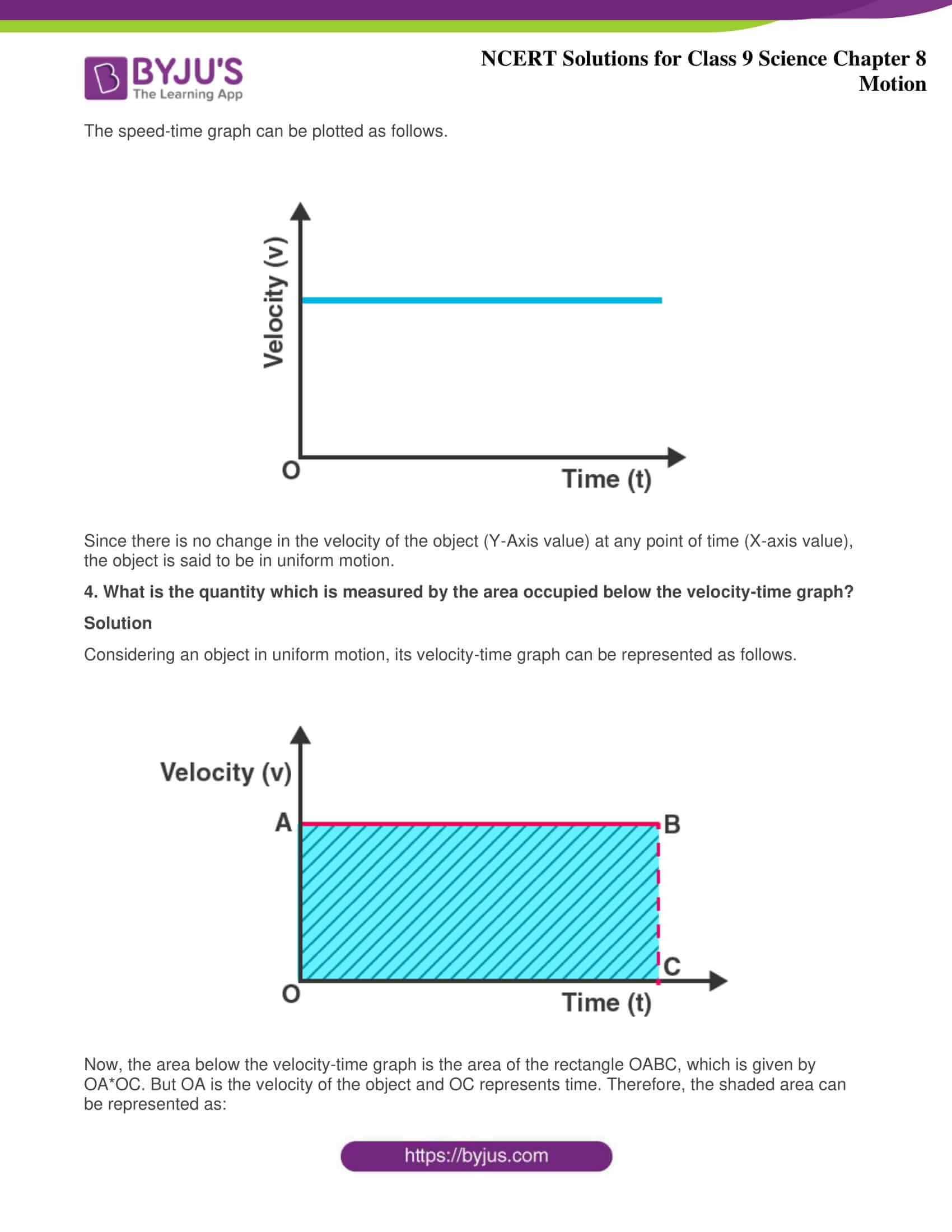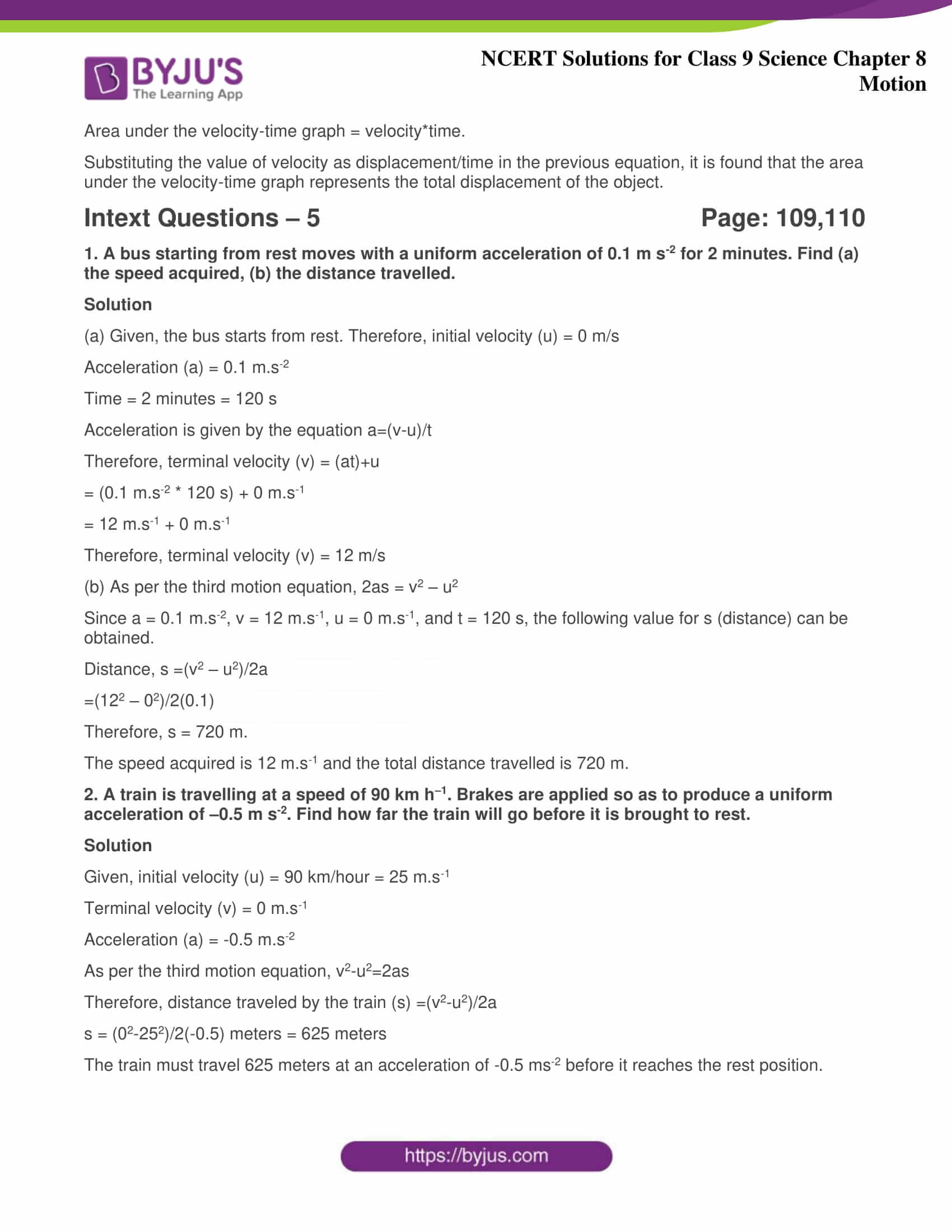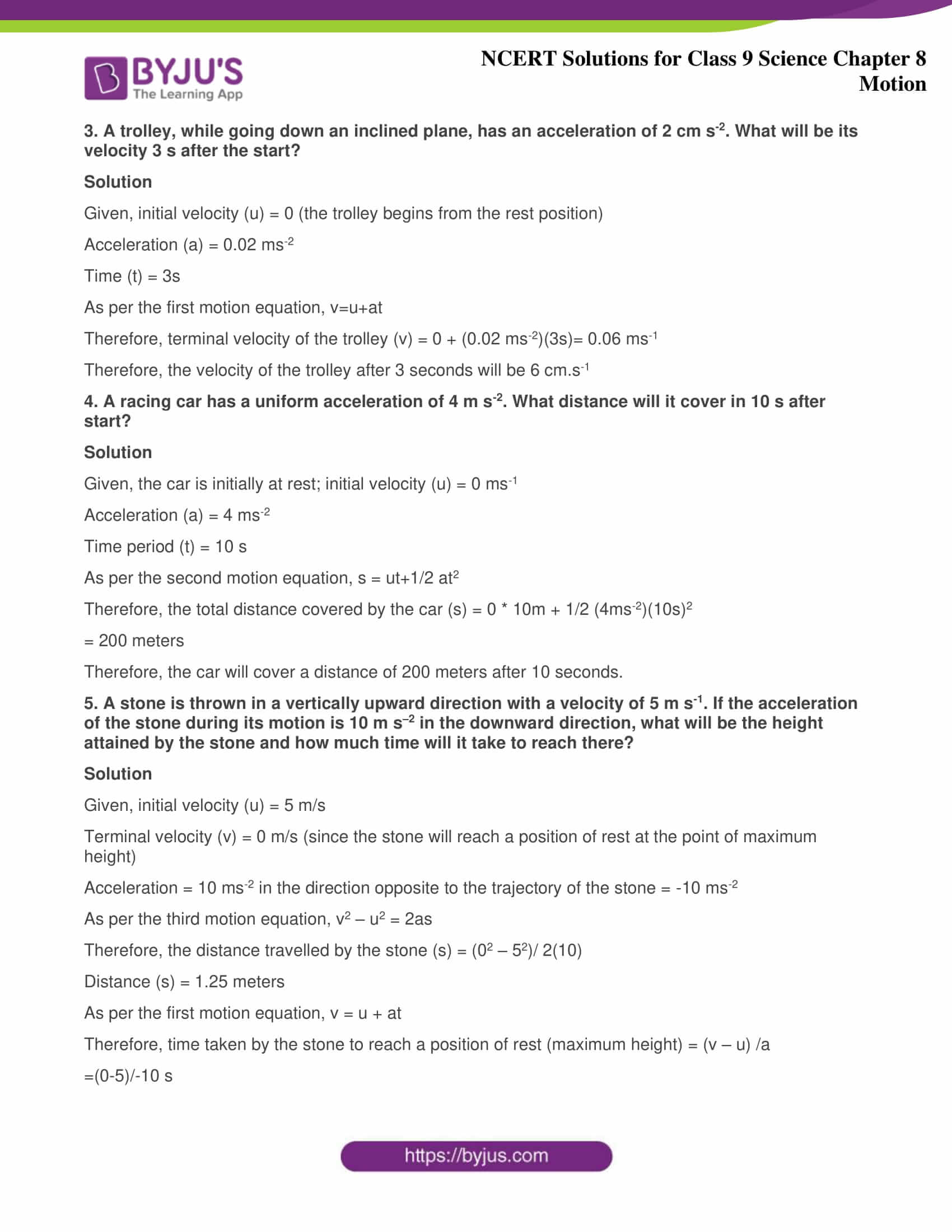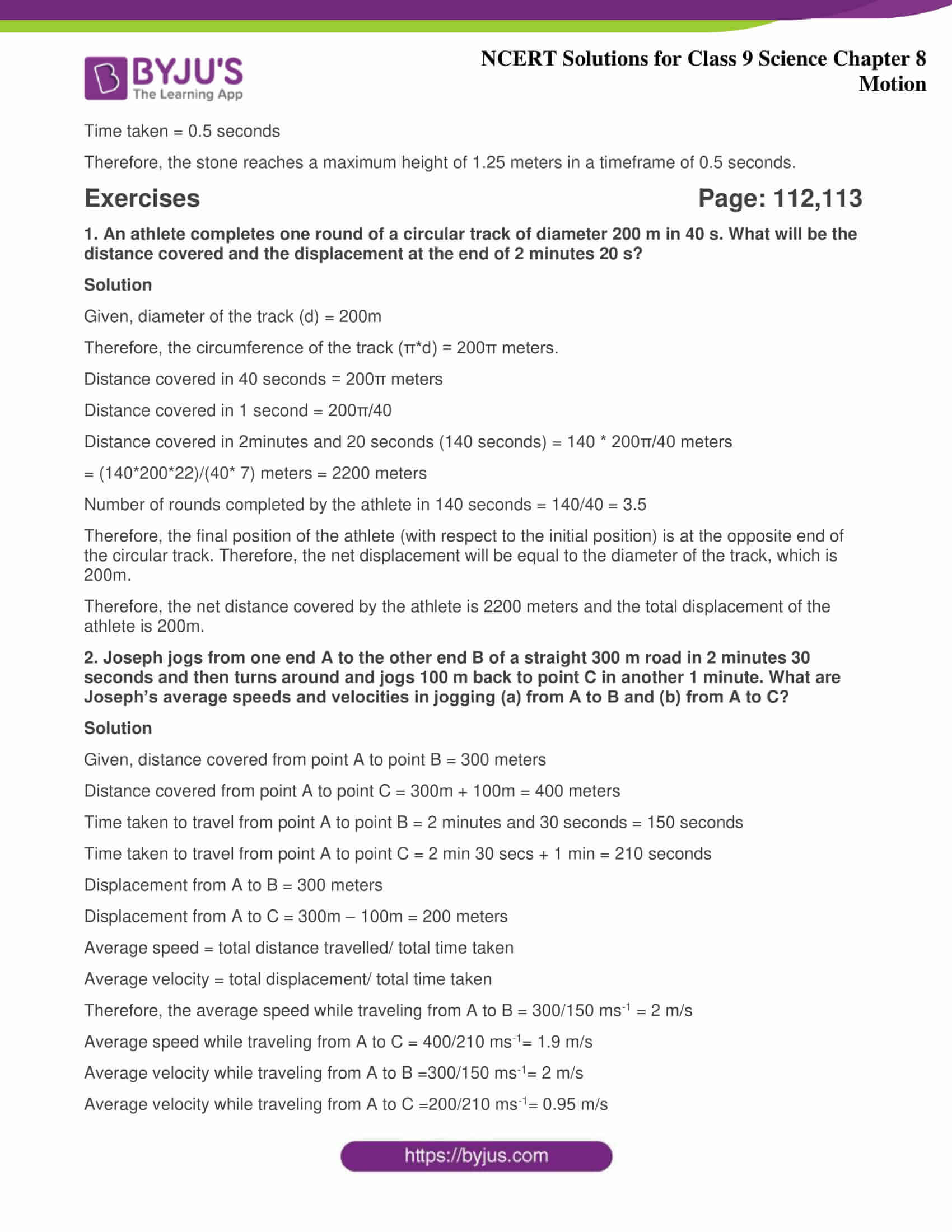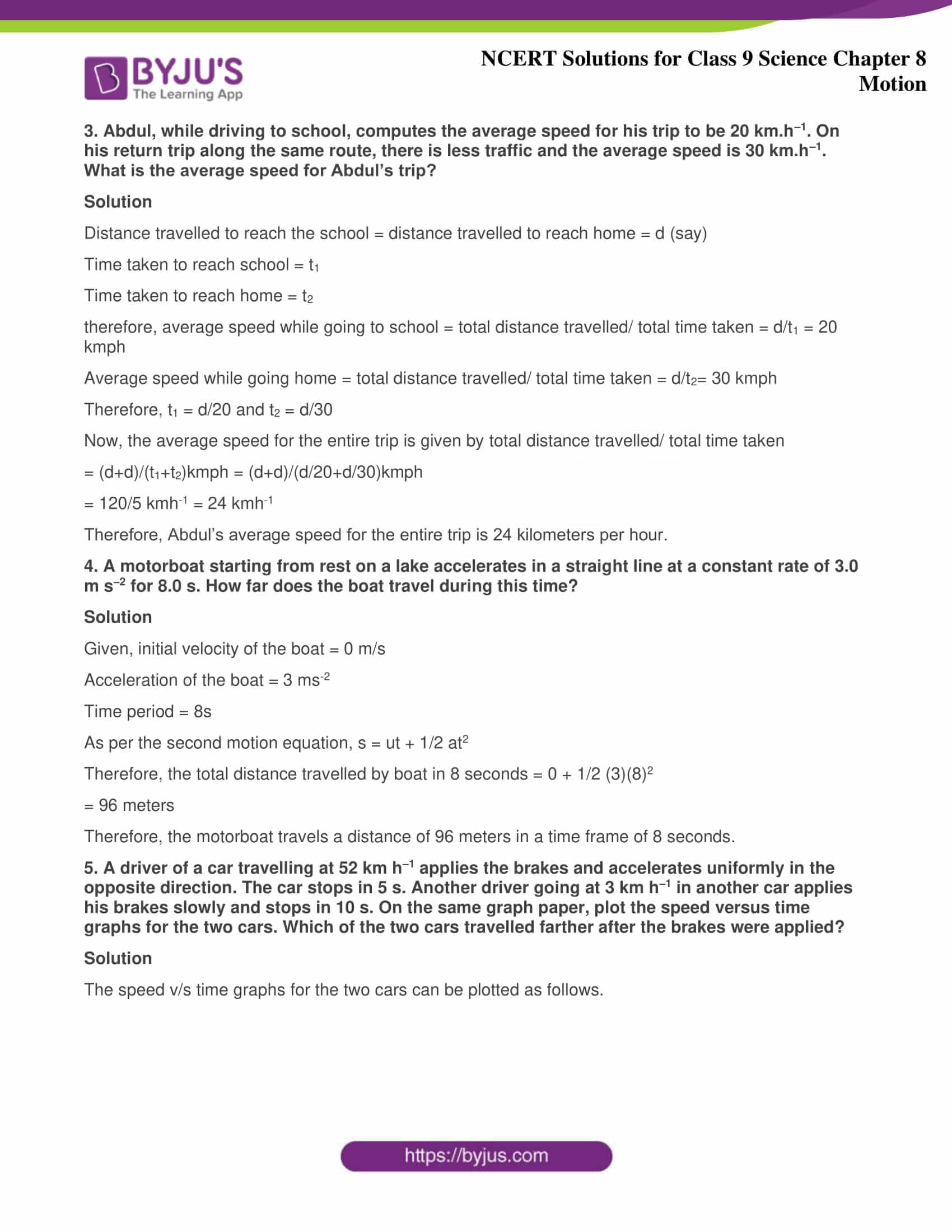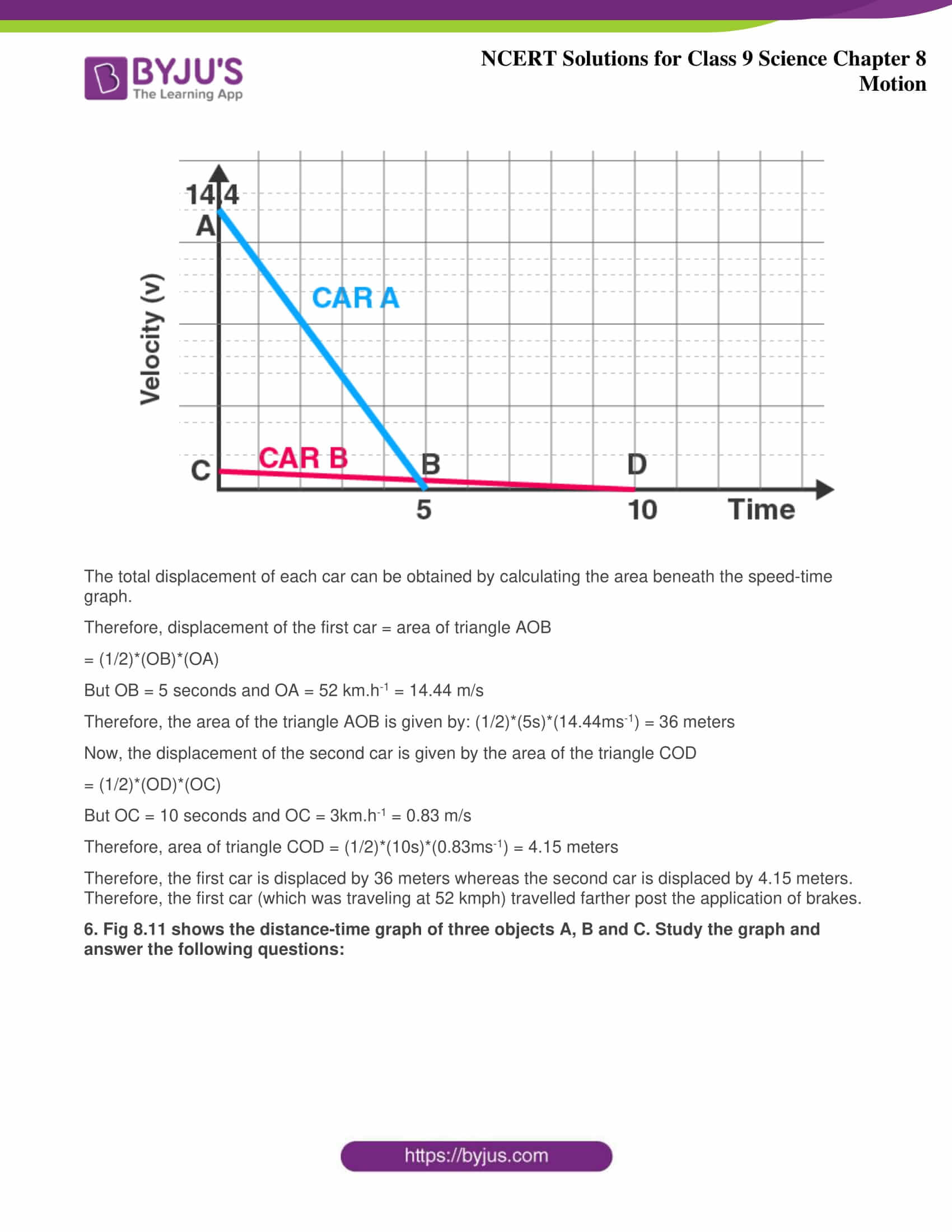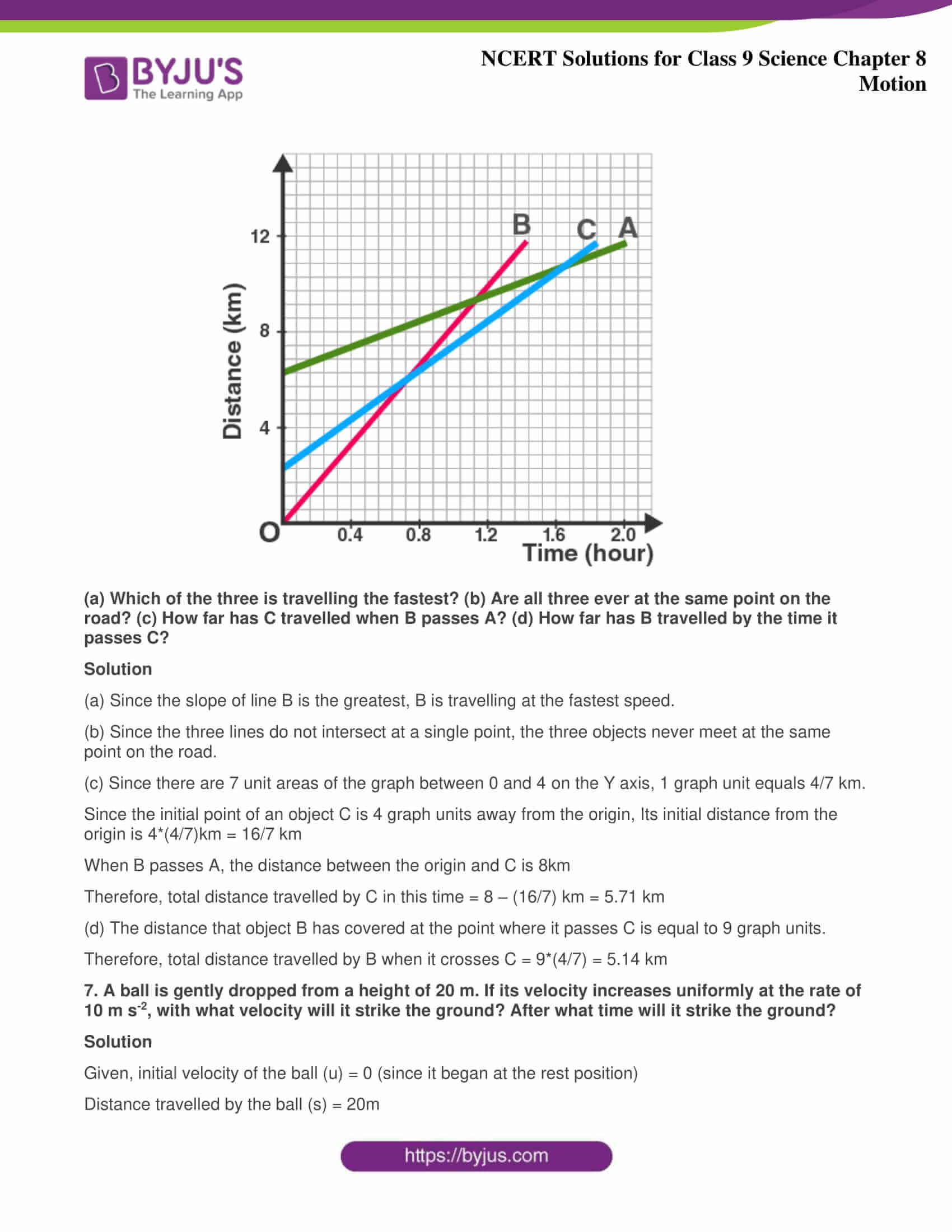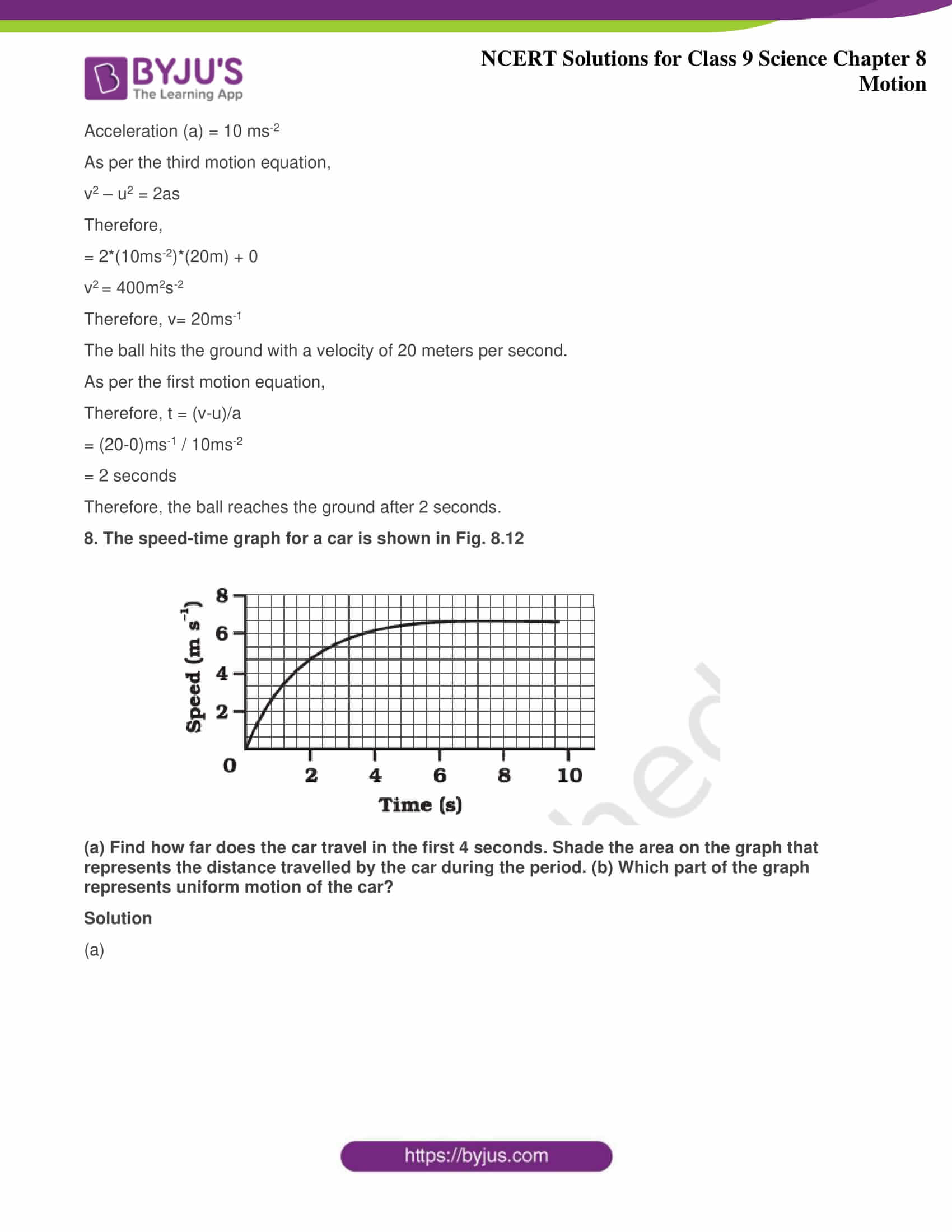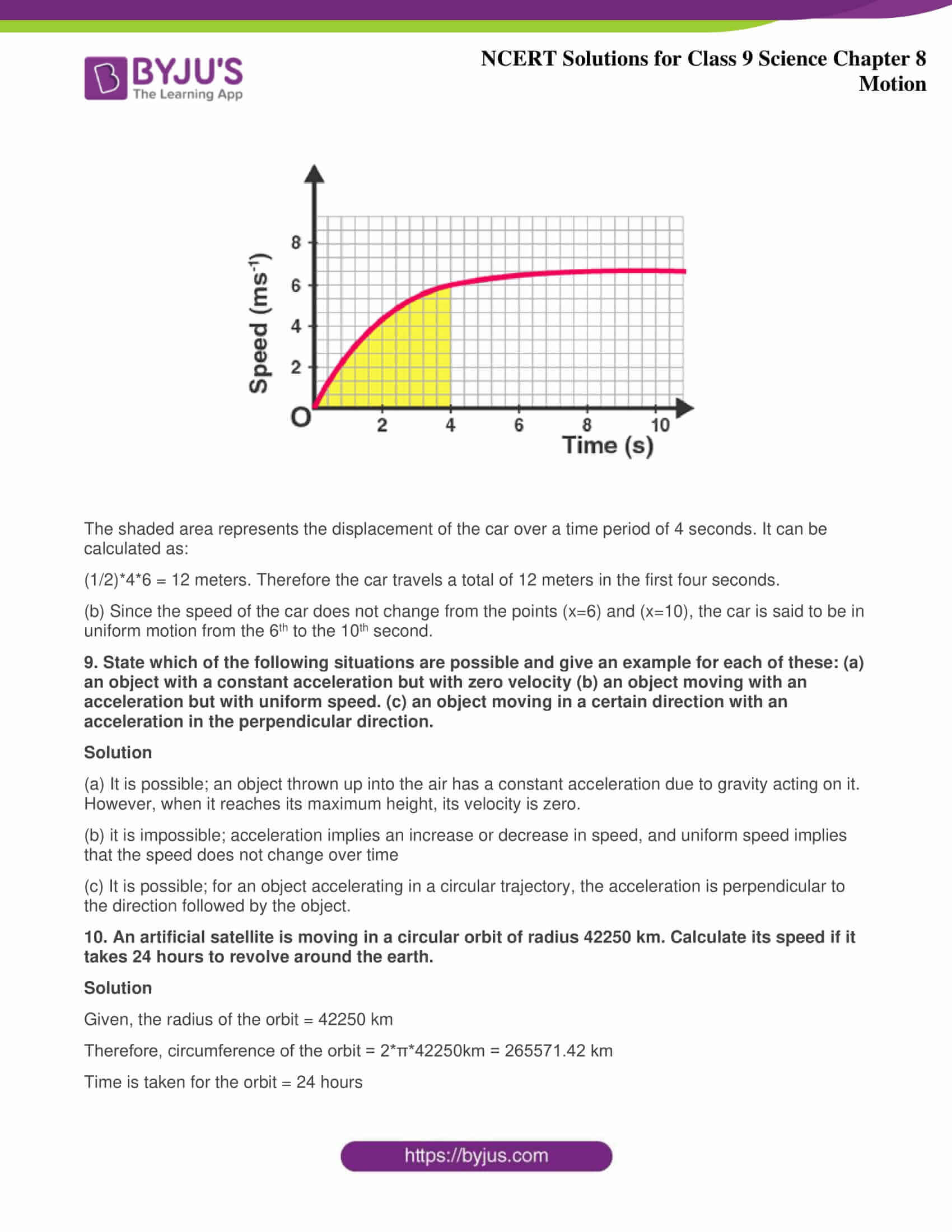### Access Answers of Science NCERT class 9 Chapter 8: Motion  (All intext and exercise questions solved)

Intext Questions - 1   Page: 100 1. An object has moved through a distance. Can it have zero displacement? If yes, support your answer with an example. Solution Yes, an object which has moved through a distance can have zero displacement if it comes back to its initial position. Example: If a person jogs in a circular park which is circular and completes one round. His initial and final position is the same. Hence, his displacement is zero. 2. A farmer moves along the boundary of a square field of side 10m in 40 s. What will be the magnitude of displacement of the farmer at the end of 2 minutes 20 seconds from his initial position? Solution Given, Side of the given square field = 10m Hence, the perimeter of a square = 40 m Time taken by the farmer  to cover the boundary of 40 m = 40 s So, in 1 s, the farmer covers a distance of 1 m Now,  Distance covered by the farmer in 2 min 20 sec = 1 x 140 = 140 m So, The total number of rotations taken by the farmer to cover a distance of 140 m = total distance/perimeter = 3.5 At this point, let us say the farmer is at point B from the origin O Therefore,  from Pythagoras theorem, the displacement s = √(102+102) s = 102 s = 14.14 m 3. Which of the following is true for displacement? (a) It cannot be zero. (b) Its magnitude is greater than the distance travelled by the object. Solution Neither of the statements is true. (a) Given statement is false because the displacement of an object which travels a certain distance and comes back to its initial position is zero. (b) Given statement is false because the displacement of an object can be equal to, but never greater than the distance travelled. Intext Questions - 2   Page: 102 1. Distinguish between speed and velocity. Solution
 Difference Between Speed and Velocity Velocity Speed Velocity can be defined as the rate at which an object changes position in a certain direction The rate at which an object covers a certain distance is known as speed The velocity of the object changes with the change in direction, therefore the object must follow one direction The average speed will continue to count even if the object changes direction Vector quantity Scalar quantity Velocity can be zero, negative, or positive Speed can never be negative or zero
2. Under what condition(s) is the magnitude of average velocity of an object equal to its average speed? Solution Since average speed is the total distance travelled in a time frame and velocity is the total displacement in the time frame, the magnitude of average velocity and average speed will be the same when the total distance travelled is equal to the displacement. 3. What does the odometer of an automobile measure? Solution An odometer, or odograph, is a device that measures the distance travelled by an automobile based on the perimeter of the wheel as the wheel rotates. 4. What does the path of an object look like when it is in uniform motion? Solution The path of an object in uniform motion is a straight line. 5. During an experiment, a signal from a spaceship reached the ground station in five minutes. What was the distance of the spaceship from the ground station? The signal travels at the speed of light, that is, 3 × 108 m/s. Solution Given that the signal travels in a straight line, the distance between the spaceship and the ground station is equal to the total distance travelled by the signal. 5 minutes = 5*60 seconds = 300 seconds. Speed of the signal = 3 × 108 m/s. Therefore, total distance = (3 × 108 m/s) * 300s = 9*1010 meters. Intext Questions - 3   Page: 103 1. When will you say a body is in (i) uniform acceleration? (ii) non-uniform acceleration? Solution Uniform Acceleration: When an object is travelling in a straight line with an increase in velocity at equal intervals of time, then the object is said to be in uniform acceleration. The free-falling of an object is an example of uniform acceleration. Non-Uniform Acceleration: When an object is travelling with an increase in velocity but not at equal intervals of time is known as non-uniform acceleration. Bus moving or leaving from the bus stop is an example of non-uniform acceleration. 2. A bus decreases its speed from 80 km h–1 to 60 km h–1 in 5 s. Find the acceleration of the bus. Solution Given, the initial velocity (u) = 80km/hour = 80000m/3600s= 22.22 m.s-1 The final velocity (v) = 60km/hour = 60000m/3600s= 16.66 m.s-1 Time frame, t = 5 seconds. Therefore, acceleration (a) =(v-u)/t = (16.66 m.s-1 - 22.22 m.s-1)/5s = -1.112 m.s-2 Therefore, the total acceleration of the bus is -1.112m.s-2. It can be noted that the negative sign indicates that the velocity of the bus is decreasing. 3. A train starting from a railway station and moving with uniform acceleration attains a speed 40 km h–1 in 10 minutes. Find its acceleration. Solution Given parameters Initial velocity (u) = 0 Final velocity (v) = 40 km/h v = 40 × (5/18) v = 11.1111 m/s Time (t) = 10 minute t = 60 x 10 t = 600 s Acceleration (a) =? Consider the formula v = u + at 11.11 = 0 + a × 600 11,11 = 600 a a = 11.11/600 a = 0.0185 ms-2 Intext Questions - 4   Page: 107 1. What is the nature of the distance-time graphs for uniform and non-uniform motion of an object? Solution For uniform motion, the distance-time graph is a straight line. On the other hand, the distance-time graph of an object in non-uniform motion is a curve.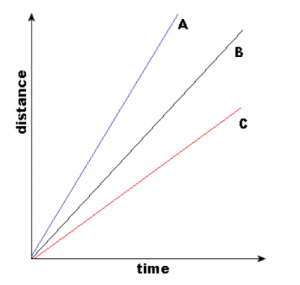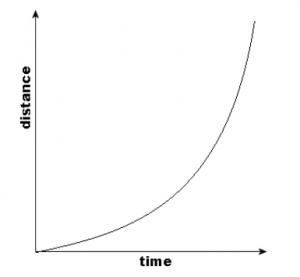The first graph describes the uniform motion and the second one describes the non-uniform motion. 2. What can you say about the motion of an object whose distance-time graph is a straight line parallel to the time axis? Solution The distance-time graph can be plotted as follows.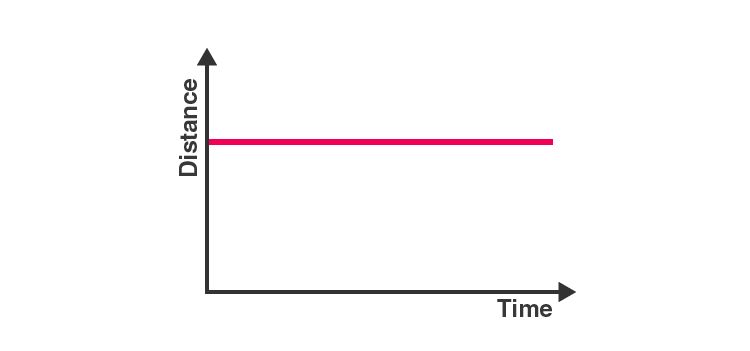When the slope of the distance-time graph is a straight line parallel to the time axis, the object is at the same position as time passes. That means the object is at rest. 3. What can you say about the motion of an object if its speed-time graph is a straight line parallel to the time axis? Solution The speed-time graph can be plotted as follows.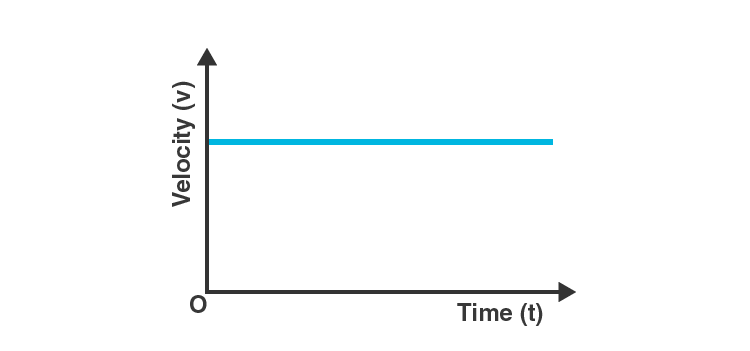Since there is no change in the velocity of the object (Y-Axis value) at any point of time (X-axis value), the object is said to be in uniform motion. 4. What is the quantity which is measured by the area occupied below the velocity-time graph? Solution Considering an object in uniform motion, its velocity-time graph can be represented as follows.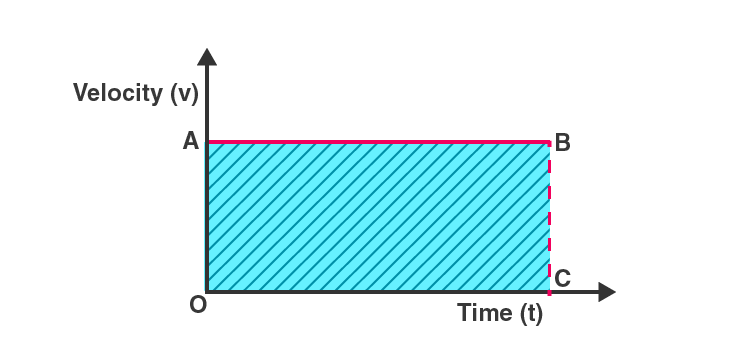Now, the area below the velocity-time graph is the area of the rectangle OABC, which is given by OA*OC. But OA is the velocity of the object and OC represents time. Therefore, the shaded area can be represented as: Area under the velocity-time graph = velocity*time. Substituting the value of velocity as displacement/time in the previous equation, it is found that the area under the velocity-time graph represents the total displacement of the object. Intext Questions - 5 Page: 109,110 1. A bus starting from rest moves with a uniform acceleration of 0.1 m s-2 for 2 minutes. Find (a) the speed acquired, (b) the distance travelled. Solution (a) Given, the bus starts from rest. Therefore, initial velocity (u) = 0 m/s Acceleration (a) = 0.1 m.s-2 Time = 2 minutes = 120 s Acceleration is given by the equation a=(v-u)/t Therefore, terminal velocity (v) = (at)+u = (0.1 m.s-2 * 120 s) + 0 m.s-1 = 12 m.s-1 + 0 m.s-1 Therefore, terminal velocity (v) = 12 m/s (b) As per the third motion equation, 2as = v2 - u2 Since a = 0.1 m.s-2, v = 12 m.s-1, u = 0 m.s-1, and t = 120 s, the following value for s (distance) can be obtained. Distance, s =(v2 - u2)/2a =(122 - 02)/2(0.1) Therefore, s = 720 m. The speed acquired is 12 m.s-1 and the total distance travelled is 720 m. 2. A train is travelling at a speed of 90 km h–1. Brakes are applied so as to produce a uniform acceleration of –0.5 m s-2. Find how far the train will go before it is brought to rest. Solution Given, initial velocity (u) = 90 km/hour = 25 m.s-1 Terminal velocity (v) = 0 m.s-1 Acceleration (a) = -0.5 m.s-2 As per the third motion equation, v2-u2=2as Therefore, distance traveled by the train (s) =(v2-u2)/2a s = (02-252)/2(-0.5) meters = 625 meters The train must travel 625 meters at an acceleration of -0.5 ms-2 before it reaches the rest position. 3. A trolley, while going down an inclined plane, has an acceleration of 2 cm s-2. What will be its velocity 3 s after the start? Solution Given, initial velocity (u) = 0 (the trolley begins from the rest position) Acceleration (a) = 0.02 ms-2 Time (t) = 3s As per the first motion equation, v=u+at Therefore, terminal velocity of the trolley (v) = 0 + (0.02 ms-2)(3s)= 0.06 ms-1 Therefore, the velocity of the trolley after 3 seconds will be 6 cm.s-1 4. A racing car has a uniform acceleration of 4 m s-2. What distance will it cover in 10 s after start? Solution Given, the car is initially at rest; initial velocity (u) = 0 ms-1 Acceleration (a) = 4 ms-2 Time period (t) = 10 s As per the second motion equation, s = ut+1/2 at2 Therefore, the total distance covered by the car (s) = 0 * 10m + 1/2 (4ms-2)(10s)2 = 200 meters Therefore, the car will cover a distance of 200 meters after 10 seconds. 5. A stone is thrown in a vertically upward direction with a velocity of 5 m s-1. If the acceleration of the stone during its motion is 10 m s–2 in the downward direction, what will be the height attained by the stone and how much time will it take to reach there? Solution Given, initial velocity (u) = 5 m/s Terminal velocity (v) = 0 m/s (since the stone will reach a position of rest at the point of maximum height) Acceleration = 10 ms-2 in the direction opposite to the trajectory of the stone = -10 ms-2 As per the third motion equation, v2 - u2 = 2as Therefore, the distance travelled by the stone (s) = (02 - 52)/ 2(10) Distance (s) = 1.25 meters As per the first motion equation, v = u + at Therefore, time taken by the stone to reach a position of rest (maximum height) = (v - u) /a =(0-5)/-10 s Time taken = 0.5 seconds Therefore, the stone reaches a maximum height of 1.25 meters in a timeframe of 0.5 seconds. Exercises Page: 112,113 1. An athlete completes one round of a circular track of diameter 200 m in 40 s. What will be the distance covered and the displacement at the end of 2 minutes 20 s? Solution Given, diameter of the track (d) = 200m Therefore, the circumference of the track (π*d) = 200π meters. Distance covered in 40 seconds = 200π meters Distance covered in 1 second = 200π/40 Distance covered in 2minutes and 20 seconds (140 seconds) = 140 * 200π/40 meters = (140*200*22)/(40* 7) meters = 2200 meters Number of rounds completed by the athlete in 140 seconds = 140/40 = 3.5 Therefore, the final position of the athlete (with respect to the initial position) is at the opposite end of the circular track. Therefore, the net displacement will be equal to the diameter of the track, which is 200m. Therefore, the net distance covered by the athlete is 2200 meters and the total displacement of the athlete is 200m. 2. Joseph jogs from one end A to the other end B of a straight 300 m road in 2 minutes 30 seconds and then turns around and jogs 100 m back to point C in another 1 minute. What are Joseph’s average speeds and velocities in jogging (a) from A to B and (b) from A to C? Solution Given, distance covered from point A to point B = 300 meters Distance covered from point A to point C = 300m + 100m = 400 meters Time taken to travel from point A to point B = 2 minutes and 30 seconds = 150 seconds Time taken to travel from point A to point C = 2 min 30 secs + 1 min = 210 seconds Displacement from A to B = 300 meters Displacement from A to C = 300m – 100m = 200 meters Average speed = total distance travelled/ total time taken Average velocity = total displacement/ total time taken Therefore, the average speed while traveling from A to B = 300/150 ms-1 = 2 m/s Average speed while traveling from A to C = 400/210 ms-1= 1.9 m/s Average velocity while traveling from A to B =300/150 ms-1= 2 m/s Average velocity while traveling from A to C =200/210 ms-1= 0.95 m/s 3. Abdul, while driving to school, computes the average speed for his trip to be 20 km.h–1. On his return trip along the same route, there is less traffic and the average speed is 30 km.h–1. What is the average speed for Abdul’s trip? Solution Distance travelled to reach the school = distance travelled to reach home = d (say) Time taken to reach school = t1 Time taken to reach home = t2 therefore, average speed while going to school = total distance travelled/ total time taken = d/t1 = 20 kmph Average speed while going home = total distance travelled/ total time taken = d/t2= 30 kmph Therefore, t1 = d/20 and t2 = d/30 Now, the average speed for the entire trip is given by total distance travelled/ total time taken = (d+d)/(t1+t2)kmph = (d+d)/(d/20+d/30)kmph = 120/5 kmh-1 = 24 kmh-1 Therefore, Abdul's average speed for the entire trip is 24 kilometers per hour. 4. A motorboat starting from rest on a lake accelerates in a straight line at a constant rate of 3.0 m s–2 for 8.0 s. How far does the boat travel during this time? Solution Given, initial velocity of the boat = 0 m/s Acceleration of the boat = 3 ms-2 Time period = 8s As per the second motion equation, s = ut + 1/2 at2 Therefore, the total distance travelled by boat in 8 seconds = 0 + 1/2 (3)(8)2 = 96 meters Therefore, the motorboat travels a distance of 96 meters in a time frame of 8 seconds. 5. A driver of a car travelling at 52 km h–1 applies the brakes and accelerates uniformly in the opposite direction. The car stops in 5 s. Another driver going at 3 km h–1 in another car applies his brakes slowly and stops in 10 s. On the same graph paper, plot the speed versus time graphs for the two cars. Which of the two cars travelled farther after the brakes were applied? Solution The speed v/s time graphs for the two cars can be plotted as follows.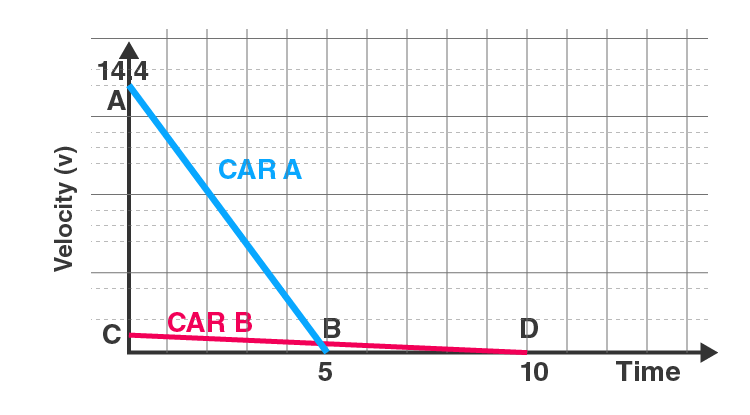The total displacement of each car can be obtained by calculating the area beneath the speed-time graph. Therefore, displacement of the first car = area of triangle AOB = (1/2)*(OB)*(OA) But OB = 5 seconds and OA = 52 km.h-1 = 14.44 m/s Therefore, the area of the triangle AOB is given by: (1/2)*(5s)*(14.44ms-1) = 36 meters Now, the displacement of the second car is given by the area of the triangle COD = (1/2)*(OD)*(OC) But OC = 10 seconds and OC = 3km.h-1 = 0.83 m/s Therefore, area of triangle COD = (1/2)*(10s)*(0.83ms-1) = 4.15 meters Therefore, the first car is displaced by 36 meters whereas the second car is displaced by 4.15 meters. Therefore, the first car (which was traveling at 52 kmph) travelled farther post the application of brakes. 6. Fig 8.11 shows the distance-time graph of three objects A, B and C. Study the graph and answer the following questions: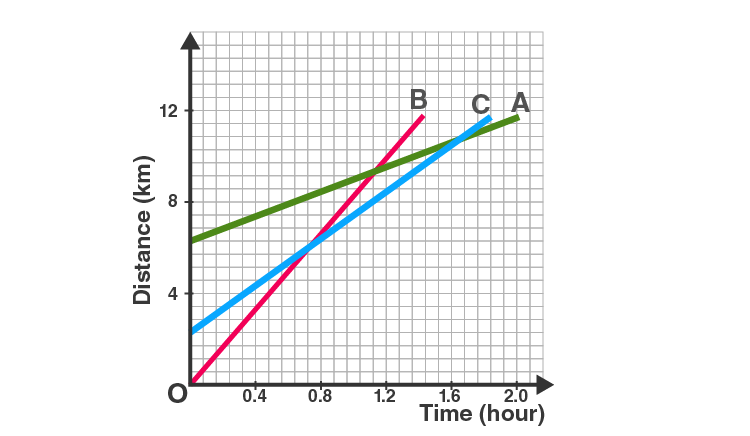(a) Which of the three is travelling the fastest? (b) Are all three ever at the same point on the road? (c) How far has C travelled when B passes A? (d) How far has B travelled by the time it passes C? Solution (a) Since the slope of line B is the greatest, B is travelling at the fastest speed. (b) Since the three lines do not intersect at a single point, the three objects never meet at the same point on the road. (c) Since there are 7 unit areas of the graph between 0 and 4 on the Y axis, 1 graph unit equals 4/7 km. Since the initial point of an object C is 4 graph units away from the origin, Its initial distance from the origin is 4*(4/7)km = 16/7 km When B passes A, the distance between the origin and C is 8km Therefore, total distance travelled by C in this time = 8 - (16/7) km = 5.71 km (d) The distance that object B has covered at the point where it passes C is equal to 9 graph units. Therefore, total distance travelled by B when it crosses C = 9*(4/7) = 5.14 km 7. A ball is gently dropped from a height of 20 m. If its velocity increases uniformly at the rate of 10 m s-2, with what velocity will it strike the ground? After what time will it strike the ground? Solution Given, initial velocity of the ball (u) = 0 (since it began at the rest position) Distance travelled by the ball (s) = 20m Acceleration (a) = 10 ms-2 As per the third motion equation, v2 - u2 = 2as Therefore, = 2*(10ms-2)*(20m) + 0 v2 = 400m2s-2 Therefore, v= 20ms-1 The ball hits the ground with a velocity of 20 meters per second. As per the first motion equation, Therefore, t = (v-u)/a = (20-0)ms-1 / 10ms-2 = 2 seconds Therefore, the ball reaches the ground after 2 seconds. 8. The speed-time graph for a car is shown in Fig. 8.12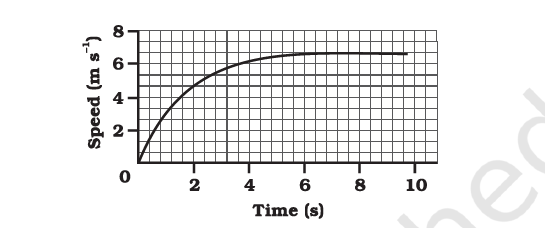(a) Find how far does the car travel in the first 4 seconds. Shade the area on the graph that represents the distance travelled by the car during the period. (b) Which part of the graph represents uniform motion of the car? Solution (a)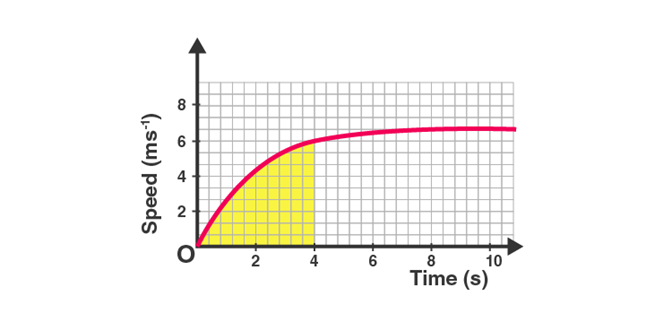The shaded area represents the displacement of the car over a time period of 4 seconds. It can be calculated as: (1/2)*4*6 = 12 meters. Therefore the car travels a total of 12 meters in the first four seconds. (b) Since the speed of the car does not change from the points (x=6) and (x=10), the car is said to be in uniform motion from the 6th to the 10th second. 9. State which of the following situations are possible and give an example for each of these: (a) an object with a constant acceleration but with zero velocity (b) an object moving with an acceleration but with uniform speed. (c) an object moving in a certain direction with an acceleration in the perpendicular direction. Solution (a) It is possible; an object thrown up into the air has a constant acceleration due to gravity acting on it. However, when it reaches its maximum height, its velocity is zero. (b) it is impossible; acceleration implies an increase or decrease in speed, and uniform speed implies that the speed does not change over time (c) It is possible; for an object accelerating in a circular trajectory, the acceleration is perpendicular to the direction followed by the object. 10. An artificial satellite is moving in a circular orbit of radius 42250 km. Calculate its speed if it takes 24 hours to revolve around the earth. Solution Given, the radius of the orbit = 42250 km Therefore, circumference of the orbit = 2*π*42250km = 265571.42 km Time is taken for the orbit = 24 hours Therefore, speed of the satellite = 11065.4 km.h-1 The satellite orbits the Earth at a speed of 11065.4 kilometers per hour.

### NCERT Solutions for Class 9 Science Chapter 8: Motion

NCERT Class 9 Science Chapter 8 explains the concept of motion, types of motion with relevant day today examples for the clear understanding of the concept. It explains the cause of the happenings of sunrise, sunset, and changing of the seasons. It helps students understand uniform and non-uniform motion. Distance-time graph and velocity-time graph, which are considered as the important concepts for examination are explained in an easy way in NCERT Solutions. It describes how the acceleration of an object is the change in velocity per unit time. NCERT Class 9 Science Chapter 8 is covered under Unit III: Motion, Force and Work and can get you maximum marks.

• NCERT Solutions for Class 9 explain motion in terms of distance moved or the displacement.
• Uniform and non-uniform motions of objects are explained through the graph and examples.
• Uniform circular motion concept is made understandable in a simple way.
• Problems on acceleration, velocity, and average velocity are also solved.

### Key Features of NCERT Solutions for Class 9 Science Chapter 8: Motion;

• The simple and easily understandable approach is followed in NCERT Solutions to make students aware of topics.
• Provides completely solved solutions to all the questions present in the respective NCERT textbooks.
• NCERT Solutions offer detailed answers to all the questions to help students in their preparations.
• These solutions will be useful for CBSE Term I exams, Science Olympiads, and other competitive exams.
[FAQ_SCHEMA_START]
Frequently Asked Questions on NCERT Solutions for Class 9 Science Chapter 8
What will I learn from the Chapter 8 of NCERT Solutions for Class 9 Science?
1. Motion in terms of distance moved or displacement is explained in this chapter. 2. Examples and graphs are provided for uniform and non uniform motions of objects. 3. The concept of uniform circular motion is explained in an understandable way. 4. Numericals based on the average velocity, velocity and acceleration are solved in a stepwise manner.
How will the NCERT Solutions for Class 9 Science Chapter 8 help us to score well in the CBSE Term I exam?
1. The NCERT Solutions follow a simple and understandable approach to make students familiar with the concepts. 2. These solutions contain answers to all the questions present in the NCERT textbook. 3. Detailed answers improve logical and analytical thinking abilities among students. 4. Students can also use these solutions to prepare for various other competitive exams along with the CBSE Term I exams.
How can I solve the problems from the NCERT Solutions for Class 9 Science Chapter 8 effortlessly?
Firstly, you have to understand the question properly and note down the data given. Important formulas and shortcut tips should be memorised to understand which formula should be substituted here. To make this easier, the experts at BYJU’S have created the NCERT Solutions with the aim of helping students score well in the CBSE Class 9 Term I exams. By regular practice, students will also be able to solve difficult problems effortlessly.
[FAQ_SCHEMA_END]